# Riemann theta-function

A superposition of theta-functions (cf. Theta-function) of the first order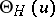,, with half-integral characteristics, and of Abelian integrals (cf. Abelian integral) of the first order, used by B. Riemann in 1857 to solve the Jacobi inversion problem.

Let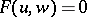be an algebraic equation which defines a compact Riemann surfaceof genus; let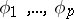be a basis of the Abelian differentials (cf. Abelian differential) of the first kind onwith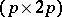-dimensional period matrix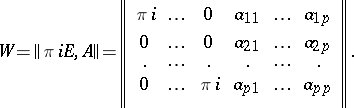Let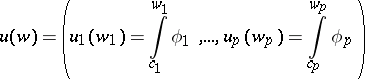be the vector of basis Abelian integrals of the first kind, where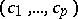is a fixed system of points inand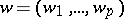is a varying system of points in. For any theta-characteristic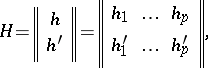where the integers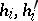take the values 0 or 1 only, it is possible to construct a theta-functionwith period matrixsuch thatsatisfies the fundamental relations(1)

Hereis the-th row vector of the identity matrix,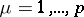. If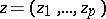is a fixed vector in the complex space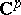, then the Riemann theta-function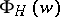can be represented as the superposition(2)

In the domain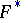that is obtained fromafter removal of sections along the cyclesof a homology basis of, the Riemann theta-functions (2) are everywhere defined and analytic. When crossing through sections the Riemann theta-functions, as a rule, are multiplied by factors whose values are determined from the fundamental relations (1). In this case, a special role is played by the theta-function of the first orderwith zero characteristic. In particular, the zeros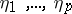of the corresponding Riemann theta-function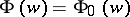determine the solution to the Jacobi inversion problem.

Quotients of Riemann theta-functions of the type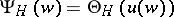with a common denominatorare used to construct analytic expressions solving the inversion problem. It can be seen from (1) that such quotients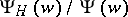can have as non-trivial factors only, and the squares of these quotients are single-valued meromorphic functions on, i.e. rational point functions on the surface. The squares and other rational functions in quotients of theta-functions used in this case are special Abelian functions (cf. Abelian function) with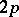periods. The specialization is expressed by the fact thatdifferent elements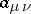of the symmetric matrix, when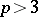, are connected by definite relations imposed by the conformal structure of, so that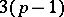remain independent among them.

Riemann theta-functions constructed for a hyper-elliptic surface, when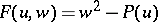where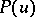is a polynomial of degree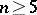without multiple roots, are sometimes referred to as hyper-elliptic theta-functions.